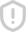### 第1章计算机，编程和Python简介习题--中文 评分1.12 将高级语言程序翻译成机器语言程序。 A.汇编程序 B.编译器 C CPU D.操作系统 答案解柝:B 第1.4节操作系统 1.13 是一个操作系统。 A. Java B.C++ C Windows xp D. Visual basic E Python 答案解析:C 1.14 是一个在计算机上运行以管理和控制计算机活动的程序 A.操作系统 B Python C.调制解调器 D.口译员 E.编译器 答案解析:A 第15节 Python的历史 1.15 Python由 创建。 A. James Gosling B.比尔盖茨 C.史蒂夫乔布斯 D. Guido van Rossum E.谷歌 答案解析 116以下哪项陈述属实? A. Python3是一个较新的版本,但它向后兼容 Python2。 B. Python3是一个较新的版本,但它不向后兼容 Python2。 C. Python2程序总是可以在 Python3解释器上运行 D. Python3程序总是可以在 Python2解释器上运行。 答案解柝:B 1.17 是一种面向对象的编程语言。 A Ja B.C++ D.C# E Python 答案解析:ABDE 被解释 A Python B.C++ D.阿达 E.帕斯卡尔 答案解析:A 第1.6节 Python入门 119要从命令提示符启动 Python,请使用命令 A execute python B run python python D go python 答案解析:C 120要运行名为tpy的 python脚本文件,请使用命令 A execute python t py B run python t. py py thon t. py D. go python t py 答案解析:C 121 Python语法区分大小写。 A是的 B.错 答案解析:A 122以下哪个代码是正确的? A print("Programming is fun") print( python is fun B print("Programming is tun") print("python is fun") print( Programming is fun) print("Python is fun") rint( Programming is fun) print("Python is fun") 答案解柝:B 第1.7节编程风格和文档 123 Python行注释以 开头。 A.∥ B/ C# D.\$ 答案解析:C 124 Python段落注释使用样式 A./评论 B./+评论木/ C."评论 D/# comments # 答案解析:C 第1.8节编程错误 1.25 错误不会导致程序中止,但会产生错误的结果。 A.语法 B.运行时 C.逻辑 答案解析:C 126在 Python中, 在处检测到语法错误。 A.编译器/编译时 B.口泽员/在运行时 C.编译器/运行时 D.口译员/编译时 答案解析:B 127以下哪个代码是正确的? print ("Programming is fun" print(" Python”) print ("Computer Science") print ("Programming is fun") print(" Python”) print ("Computer Science") print( programming is fun") print("Python) print("Computer Science") print("Programming is fun ") print("python print(computer Science")A. B.‖ C D.Ⅳ 答案解析:D 第19节图形编程入门 128要显示乌龟对象的当前位置和方向,请使用 A turtle. show O B turtle. showLocation O C. turtle. show Direction o D. turtle. showturtle () E turtle showTurtle 答案解析:D 129要将乌龟移动到(4,5)处,请使用 A turtle. move(4,5) B turtle moveTo(4, 5) C. turtle. moveto (4.5) D. turtle go (4, 5) Eturtle. goto(4, 5) 答案解析:E 130要绘制半径为50的圆,请使用 A turtle circle (50) B turtle circle (100) C. turtle drawcircle (50) D. turtle. draw Circle (50) 答案解析:A 131要抬起笔,请使用 A turtle penup o B turtle penup C. turtle. lift D. turtle. up 答案解析:B 132要放下笔,请使用 A turtle pen Down O) B. turtle pendown O C turtle putDown O D. turtle down () 答案解析:B

...展开详情举报收藏分享William chu